Adjacent Angles, Linear Pair of angles, Vertically Opposite angles

Chapter 5 Class 7 Lines and Angles
Concept wise

Adjacent angles are angles that are side by side.

• Common vertex
• Common side
• No overlap of angles

Let’s look at some examples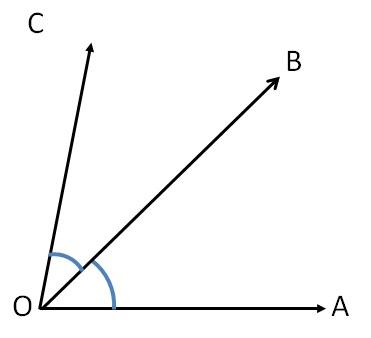Here, the angles are adjacent as

• They have common vertex O
• They have common side OB
• There is no overlap

### Are the angles adjacent to each other?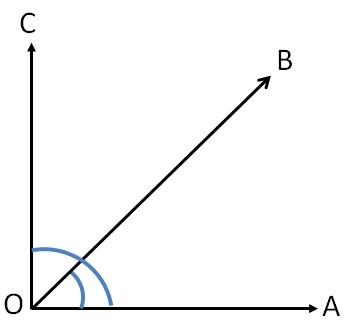Hence angles are overlapping each other

So, they are not adjacent angles

### Are the angles adjacent to each other?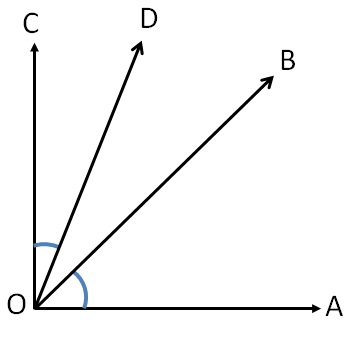Hence, the angles do not share a common side

So, they are not adjacent angles

### Are the angles adjacent to each other?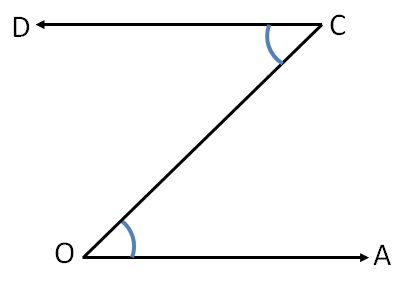Hence, the angles do not share a common vertex

So, they are not adjacent angles

### Are the angles adjacent to each other?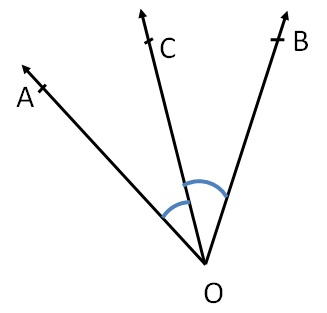Here,

• Angles have a common vertex O,
• Angles have a common side OC,
• And there is no overlap of angles.

### Are the angles adjacent to each other?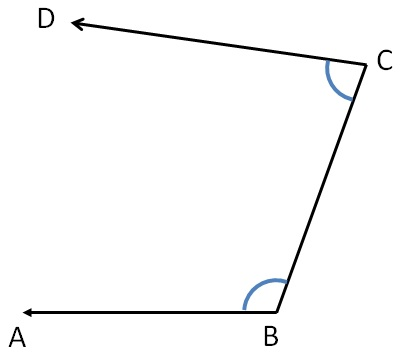Here,

Angles do not have a common vertex

∴ They are not adjacent angles

### Are the angles adjacent to each other?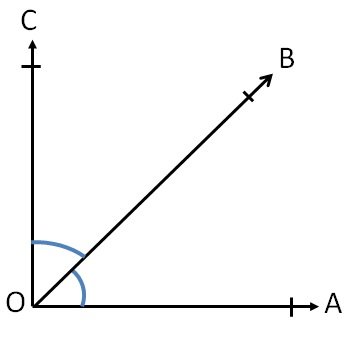Here,

• Angles have a common vertex O,
• They have a common side OB and
• There is no overlap of angles

### Are the angles adjacent to each other?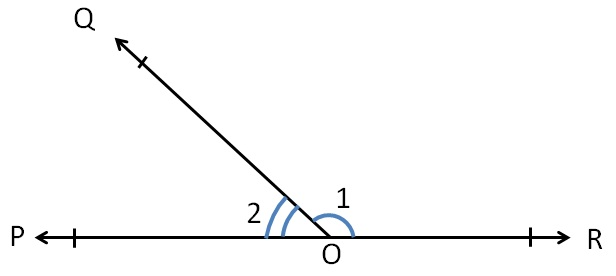Here,

• Angles have a common vertex O,
• They have a common side OB and
• There is no overlap of angles

### Are the angles adjacent to each other?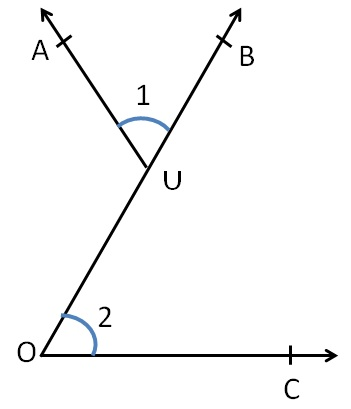Here,

Angles do no have a common vertex

∴ They are not adjacent angles

### Are the angles adjacent to each other?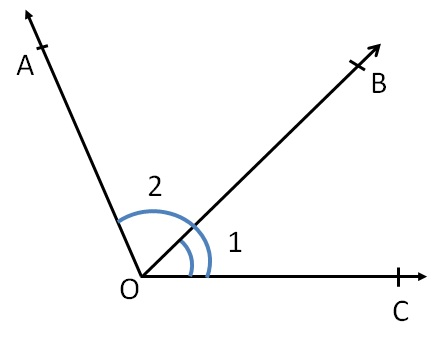Here,

Angles are overlapping each others

So, they are not adjacent angles

### Are the angles adjacent to each other?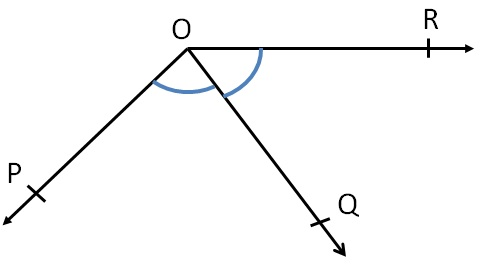Here,

• Angles have a common vertex O,
• They have a common side OQ and
• There is no overlap of angles

### Are the angles adjacent to each other?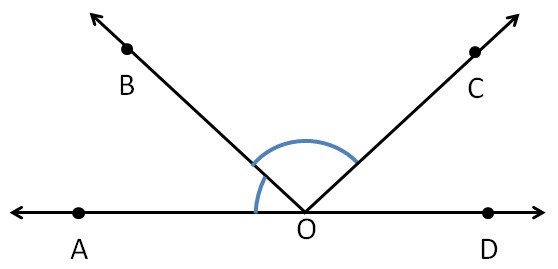Here,

• Angles have a common vertex O,
• They have a common side OB and
• There is no overlap of angles

### Are the angles adjacent to each other?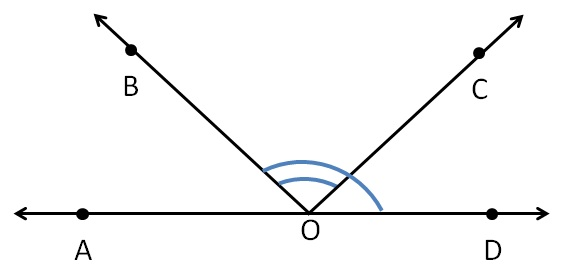Here,

Angles are overlapping each others

So, they are not adjacent angles.

Get live Maths 1-on-1 Classs - Class 6 to 12